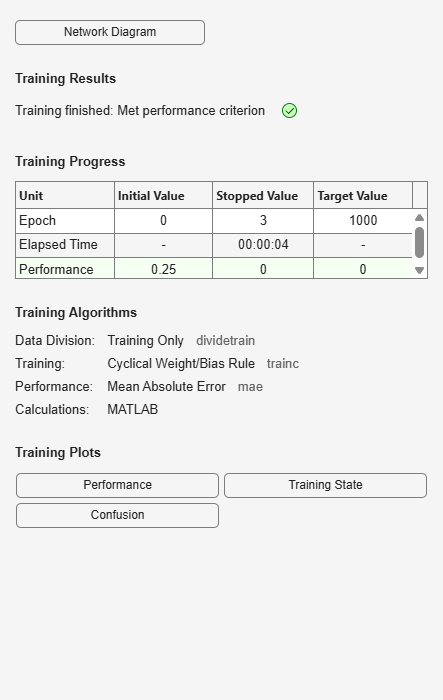# perceptron

Simple single-layer binary classifier

## Syntax

``perceptron(hardlimitTF,perceptronLF)``

## Description

example

``` NoteDeep Learning Toolbox™ supports perceptrons for historical interest. For better results, you should instead use `patternnet`, which can solve nonlinearly separable problems. Sometimes the term “perceptrons” refers to feed-forward pattern recognition networks; but the original perceptron, described here, can solve only simple problems. `perceptron(hardlimitTF,perceptronLF)` takes a hard limit transfer function, `hardlimitTF`, and a perceptron learning rule, `perceptronLF`, and returns a perceptron.In addition to the default hard limit transfer function, perceptrons can be created with the `hardlims` transfer function. The other option for the perceptron learning rule is `learnpn`.Perceptrons are simple single-layer binary classifiers, which divide the input space with a linear decision boundary.Perceptrons can learn to solve a narrow range of classification problems. They were one of the first neural networks to reliably solve a given class of problem, and their advantage is a simple learning rule.```

## Examples

collapse all

This example shows how to use a perceptron to solve a simple classification logical-OR problem.

```x = [0 0 1 1; 0 1 0 1]; t = [0 1 1 1]; net = perceptron; net = train(net,x,t); view(net) y = net(x); ```## Input Arguments

collapse all

Hard limit transfer function.

Perceptron learning rule.